# NCERT solutions for Class 7 Maths chapter 7 - Congruence of Triangles [Latest edition]

#### Chapters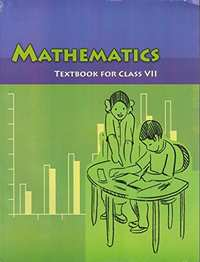## Chapter 7: Congruence of Triangles

Exercise 7.1Exercise 7.2
Exercise 7.1 [Page 137]

### NCERT solutions for Class 7 Maths Chapter 7 Congruence of TrianglesExercise 7.1 [Page 137]

Exercise 7.1 | Q 1.1 | Page 137

Complete the following statements:

Two line segments are congruent if __________.

Exercise 7.1 | Q 1.2 | Page 137

Complete the following statements:

Among two congruent angles, one has a measure of 70°; the measure of the other angle is __________.

Exercise 7.1 | Q 1.3 | Page 137

Complete the following statements:

When we write ∠A = ∠ B, we actually mean __________

Exercise 7.1 | Q 2 | Page 137

Give any two real-life examples for congruent shapes.

Exercise 7.1 | Q 3 | Page 137

If ΔABC ≅ ΔFED under the correspondence ABC ↔ FED, write all the Corresponding congruent parts of the triangles.

Exercise 7.1 | Q 4.1 | Page 137

If ΔDEF ≅ ΔBCA, write the part(s) of ΔBCA that correspond to ∠E

Exercise 7.1 | Q 4.2 | Page 137

If ΔDEF ≅ ΔBCA, write the part(s) of ΔBCA that correspond to bar(EF)

Exercise 7.1 | Q 4.3 | Page 137

If ΔDEF ≅ ΔBCA, write the part(s) of ΔBCA that correspond to ∠F

Exercise 7.1 | Q 4.4 | Page 137

If ΔDEF ≅ ΔBCA, write the part(s) of ΔBCA that correspond to bar(DF)

Exercise 7.2 [Pages 149 - 151]

### NCERT solutions for Class 7 Maths Chapter 7 Congruence of TrianglesExercise 7.2 [Pages 149 - 151]

Exercise 7.2 | Q 1 | Page 149

Which congruence criterion do you use in the following?

Given: AC = DF

AB = DE

BC = EF

So, ΔABC ≅ ΔDEF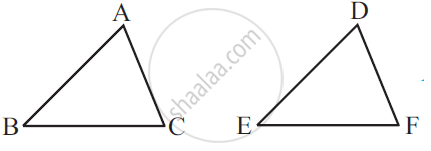Exercise 7.2 | Q 1.2 | Page 149

Which congruence criterion do you use in the following?

Given: ZX = RP

RQ = ZY

∠PRQ = ∠XZY

So, ΔPQR ≅ ΔXYZ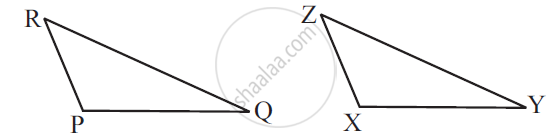Exercise 7.2 | Q 1.3 | Page 149

Which congruence criterion do you use in the following?

Given: ∠MLN = ∠FGH

∠NML = ∠GFH

ML = FG

So, ΔLMN ≅ ΔGFH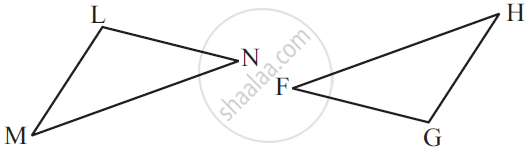Exercise 7.2 | Q 1.4 | Page 149

Which congruence criterion do you use in the following?

Given: EB = DB

AE = BC

∠A = ∠C = 90°

So, ΔABE ≅ ΔCDB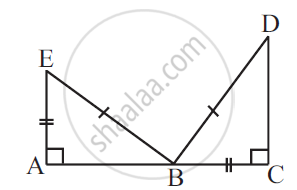Exercise 7.2 | Q 2.1 | Page 149

You want to show that ΔART ≅ ΔPEN,

If you have to use SSS criterion, then you need to show

1) AR =

2) RT =

3) AT =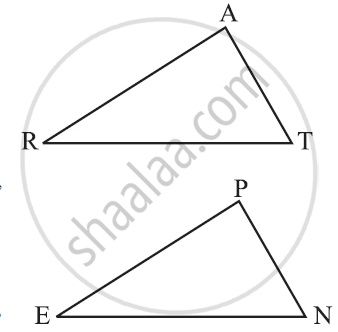Exercise 7.2 | Q 2.2 | Page 149

You want to show that ΔART ≅ ΔPEN,

If it is given that ∠T = ∠N and you are to use SAS criterion, you need to have

1) RT = and

2) PN =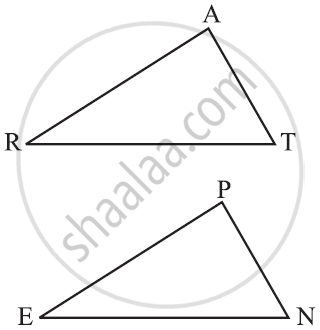Exercise 7.2 | Q 2.3 | Page 149

You want to show that ΔART ≅ ΔPEN,

If it is given that AT = PN and you are to use ASA criterion, you need to have

1) ?

2) ?Exercise 7.2 | Q 3 | Page 150

You have to show that ΔAMP ≅ AMQ.

In the following proof, supply the missing reasons.

 Steps Reasons 1 PM = QM 1 ... 2 ∠PMA = ∠QMA 2 ... 3 AM = AM 3 ... 4 ΔAMP ≅ ΔAMQ 4 ...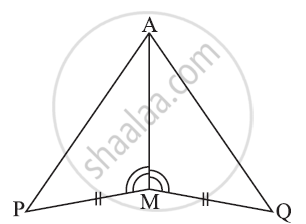Exercise 7.2 | Q 4 | Page 150

In ΔABC, ∠A = 30°, ∠B = 40° and ∠C = 110°

In ΔPQR, ∠P = 30°, ∠Q = 40° and ∠R = 110°

A student says that ΔABC ≅ ΔPQR by AAA congruence criterion. Is he justified? Why or why not?

Exercise 7.2 | Q 5 | Page 150

In the figure, the two triangles are congruent.

The corresponding parts are marked. We can write ΔRAT ≅ ?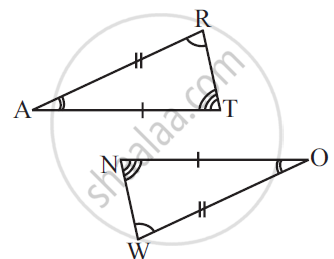Exercise 7.2 | Q 6 | Page 150

Complete the congruence statement:

ΔBCA ≅?

ΔQRS ≅?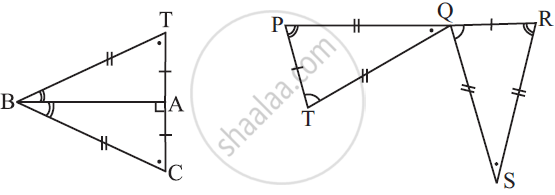Exercise 7.2 | Q 7.1 | Page 150

In a squared sheet, draw two triangles of equal areas such that

The triangles are congruent.

What can you say about their perimeters?

Exercise 7.2 | Q 7.2 | Page 150

In a squared sheet, draw two triangles of equal areas such that

The triangles are not congruent.

What can you say about their perimeters?

Exercise 7.2 | Q 9 | Page 150

If ΔABC and ΔPQR are to be congruent, name one additional pair of corresponding parts. What criterion did you use?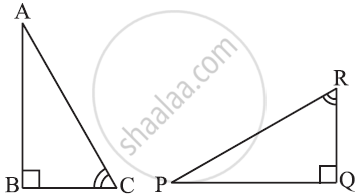Exercise 7.2 | Q 10 | Page 151

Explain, why ΔABC ≅ ΔFED.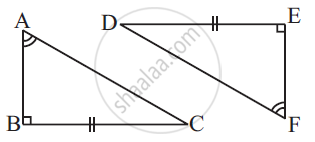## Chapter 7: Congruence of Triangles

Exercise 7.1Exercise 7.2## NCERT solutions for Class 7 Maths chapter 7 - Congruence of Triangles

NCERT solutions for Class 7 Maths chapter 7 (Congruence of Triangles) include all questions with solution and detail explanation. This will clear students doubts about any question and improve application skills while preparing for board exams. The detailed, step-by-step solutions will help you understand the concepts better and clear your confusions, if any. Shaalaa.com has the CBSE Class 7 Maths solutions in a manner that help students grasp basic concepts better and faster.

Further, we at Shaalaa.com provide such solutions so that students can prepare for written exams. NCERT textbook solutions can be a core help for self-study and acts as a perfect self-help guidance for students.

Concepts covered in Class 7 Maths chapter 7 Congruence of Triangles are Congruence of Plane Figures, Congruence Among Line Segments, Congruence of Angles, Congruence of Triangles, Criteria for Congruence of Triangles, RHS Congruence Criterion, SSS Congruence Criterion, SAS Congruence Criterion, ASA Congruence Criterion, Exceptional Criteria for Congruence of Triangles.

Using NCERT Class 7 solutions Congruence of Triangles exercise by students are an easy way to prepare for the exams, as they involve solutions arranged chapter-wise also page wise. The questions involved in NCERT Solutions are important questions that can be asked in the final exam. Maximum students of CBSE Class 7 prefer NCERT Textbook Solutions to score more in exam.

Get the free view of chapter 7 Congruence of Triangles Class 7 extra questions for Class 7 Maths and can use Shaalaa.com to keep it handy for your exam preparation